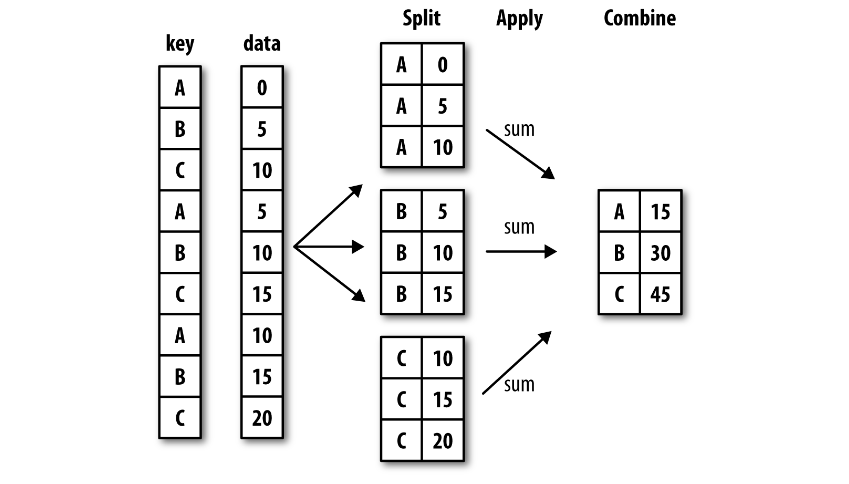# 4.4 - Grouping and Aggregation

Last updated: November 25th, 2019# Grouping and Aggregation¶

An essential piece of analysis of large data is efficient summarizaion: computing aggregations like sum(), mean(), min(), max(), in which a single number gives insight into the nature of a potentially large dataset.## Hands on!¶

In [ ]:
import pandas as pd
import numpy as np### NBA players data¶

We'll use a small NBA dataset which gives information about its players.

In [ ]:
players = pd.DataFrame({
'salary': [
33285709, 31269231, 34682550, 25000000, 17826150,
29512900, 28530608, 26243760, 18868625, 2500000
],
'season_start': [2017, 2017, 2017, 2017, 2017, 2017, 2017, 2017, 2017, 2017],
'season_end': [2018, 2018, 2018, 2018, 2018, 2018, 2018, 2018, 2018, 2018],
'team': ['CLE', 'DEN', 'GSW', 'GSW', 'GSW', 'LAC', 'OKC', 'OKC', 'SAS', 'SAS'],
'Pos': ['SF', 'PF', 'PG', 'PF', 'SG', 'PF', 'PG', 'SF', 'SF', 'SG'],
'Age': [32.0, 31.0, 28.0, 28.0, 26.0, 27.0, 28.0, 32.0, 25.0, 39.0]
}, index=[
'LeBron James', 'Paul Millsap', 'Stephen Curry', 'Kevin Durant',
'Klay Thompson', 'Blake Griffin', 'Russell Westbrook',
'Carmelo Anthony', 'Kawhi Leonard', 'Manu Ginobili'
])

In [ ]:
players.sort_index(inplace=True)

In [ ]:
players

In [ ]:
players.info()### Understanding Grouping¶

We can manually simulate the Split > Apply > Combine operation.

#### 1. Split (split players by positions)¶

This step involves breaking up and grouping a DataFrame depending on the value of the specified key.

In [ ]:
players['Pos'].value_counts()


Position = 'PF'

In [ ]:
players[players['Pos'] == 'PF']


Position = 'SF'

In [ ]:
players[players['Pos'] == 'SF']


Position = 'PG'

In [ ]:
players[players['Pos'] == 'PG']


#### 2. Apply (apply a function to each group)¶

This step involves computing some function, usually an aggregate, transformation, or filtering, within the individual groups.

For example, get the max salary per group (per position):

In [ ]:
top_PF_salary = players[players['Pos'] == 'PF'].sort_values(by='salary', ascending=False)

top_PF_salary

In [ ]:
top_SF_salary = players[players['Pos'] == 'SF'].sort_values(by='salary', ascending=False)

top_SF_salary

In [ ]:
top_PG_salary = players[players['Pos'] == 'PG'].sort_values(by='salary', ascending=False)

top_PG_salary


#### 3. Combine¶

This step merges the results of these operations into an output array.

In [ ]:
pd.concat([top_PF_salary, top_SF_salary, top_PG_salary])### The GroupBy object¶

We can compute the most basic split-apply-combine operation with the groupby() method of DataFrames, passing the name of the desired key column:

In [ ]:
players['salary'].groupby(players['Pos'])### Aggregation¶

The GroupBy object does no actual computation until the aggregation is applied to it. To produce a result, we can apply an aggregate to that object which will perform the appropiate apply/combine steps to produce the desired result.

This is usually referred to as an aggregation, you have a set of values (for example, all the salaries of the players in position PG) and you want to "aggregate" them or "reduce" them to a single value that has meaning for you. In this case, the aggregation is the max function. For each group of players (split by position), we want to get the max salary:

In [ ]:
players['salary'].groupby(players['Pos']).max().to_frame()


The max function in this case, is the applied function, we could apply any other different function, for example, mean to get the average salary per position:

In [ ]:
players['salary'].groupby(players['Pos']).mean().to_frame()

In [ ]:
players['salary'].groupby(players['Pos']).describe()


We could verify it works manually:

In [ ]:
players[players['Pos'] == 'PG'].sort_values(by='salary', ascending=False)

In [ ]:
players.loc[players['Pos'] == 'PG', 'salary'].mean()


The result of the groupby operation is a Series, indexed by the group and containing the aggregation result as the values. What happens if you want to group by multiple parameters?

In [ ]:
players['salary'].groupby([players['team'], players['Pos']]).max().to_frame()


The result is also a Series, but with a hierarchical index.

In the case of grouping by multiple features, order matters. Changing the order of the keys to use to group will alter the resulting Series:

In [ ]:
players['salary'].groupby([players['Pos'], players['team']]).max().to_frame()


Although the values will need to match in the common keys. For example, the max salary of "Team GSW, position PF" is the same as max salary of "position PF, team GSW".### Custom aggregations¶

Most common aggregation functions are already provided (min, max, mean, etc.). But you can also use other custom functions with the aggregate method:

In [ ]:
players.groupby([players['team']]).aggregate(np.sum)


As you can see, aggregate is running the function in every column, even in those that don't make sense (like season_end or season_start). We can, of course, select the columns to use beforehand:

In [ ]:
players[['salary', 'Age']].groupby([players['team']]).aggregate(np.max)


Or...

In [ ]:
players.groupby([players['team']])[['salary', 'Age']].aggregate(np.max)


#### aggregate and multiple parameters¶

Grouping by multiple parameters also works with aggregate:

In [ ]:
players[['salary', 'Age']].groupby([players['team'], players['Pos']]).aggregate(np.max)


#### Multiple functions¶

aggregate can also take multiple functions to apply to the groups:

In [ ]:
def range_max_min(values):
return values.max() - values.min()

In [ ]:
players[['salary', 'Age']].groupby([players['team']]).aggregate([np.max, np.min, range_max_min])


#### Dict of operations¶

You can also pass to aggregate a dictionary containing different operations to perform, based on the column:

In [ ]:
players[['salary', 'Age']].groupby([players['team']]).aggregate({
'salary': np.max,
'Age': np.mean
})### The apply() method¶

apply is also a method available to run custom operations on your groups. It's similar to aggregate, so we usually recommend the later one over apply:

In [ ]:
players['salary'].groupby([players['team']]).apply(np.max).to_frame()

In [ ]:
players['salary'].groupby([players['team']]).apply(lambda x: x.max() - x.min()).to_frame()### More examples using the whole data¶

Now we'll use the complete dataset and try more grouping and aggregations

In [ ]:
df = pd.read_csv('data/nba_2017_cleaned.csv', index_col=1, dtype={
'Tm': 'category',
'Pos': 'category'
})

In [ ]:
df.head()

In [ ]:
df.info()

In [ ]:
df['FG'].groupby(df['Pos']).mean()

In [ ]:
df.groupby('Pos').mean()

In [ ]:
df['FG'].groupby(df['Pos']).size().to_frame()

In [ ]:
df.groupby('Pos').size().to_frame()


We can also pass custom functions:

In [ ]:
df['FG'].groupby(df['Pos']).apply(np.mean).to_frame()